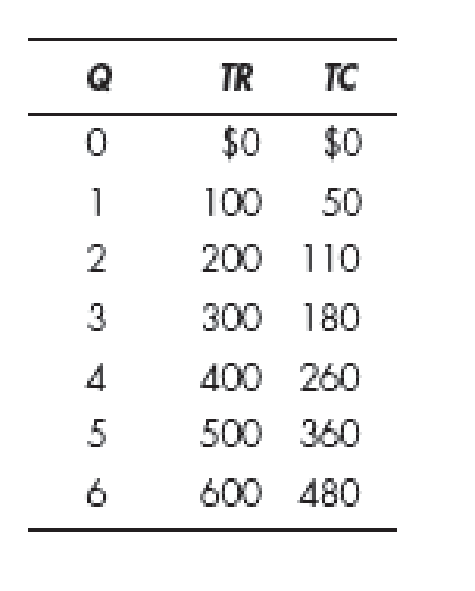Chapter 9, Problem 3WNG

Chapter
Section
Textbook Problem

According to the accompanying table, what quantity of output should the firm produce? Explain your answer.To determine

Quantity of output produced by the firm.

Explanation

Table 1 shows the different quantities of output produced at different total revenues and total costs.

Table 1

 Quantity Total revenue Total cost 0 $0$0 1 100 50 2 200 110 3 300 180 4 400 260 5 500 360 6 600 480

From the different quantitates shown in Table 1, the firm should produce 5 units of output because the total revenue is greater than the total cost, and also at quantity 5, the marginal revenue is equal to the marginal cost. The calculation of marginal revenue and marginal cost is shown below:

Marginal revenue can be calculated as follows:

Marginal revenue=Total revenuePresentTotal revenuePreviousQuantityPresentQuantityPrevious        (1)

Substitute the respective values in Equation (1) to calculate the marginal revenue at quantity 5 units

Still sussing out bartleby?

Check out a sample textbook solution.

See a sample solution

The Solution to Your Study Problems

Bartleby provides explanations to thousands of textbook problems written by our experts, many with advanced degrees!

Get Started

What is inflation and what causes it?

Principles of Microeconomics (MindTap Course List)

Identify three measures of liquidity calculated by FCI.

College Accounting, Chapters 1-27 (New in Accounting from Heintz and Parry)

Explain how a trial balance and a balance sheet differ.

College Accounting (Book Only): A Career Approach

What are economic resources?

Accounting Information Systems

EXPECTATIONS THEORY Interest rates on 4-year Treasury securities are currently 7%, while 6-year Treasury securi...

Fundamentals of Financial Management, Concise Edition (with Thomson ONE - Business School Edition, 1 term (6 months) Printed Access Card) (MindTap Course List)